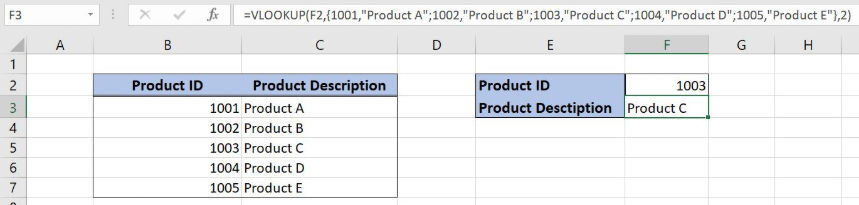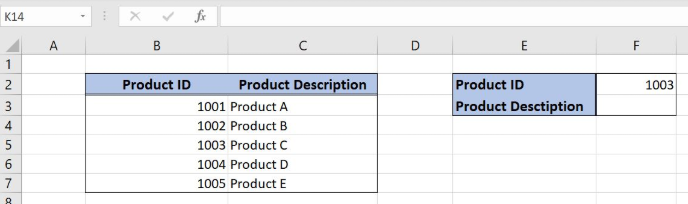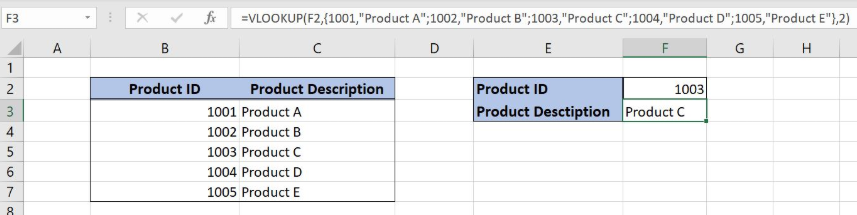Get instant live expert help with Excel or Google Sheets“My Excelchat expert helped me in less than 20 minutes, saving me what would have been 5 hours of work!”

#### Post your problem and you’ll get Expert help in seconds.

Your message must be at least 40 characters
Our professional Expert are available now. Your privacy is guaranteed.

# Self-contained VLOOKUP

Excel allows a user to specify a self-contained lookup table for VLOOKUP function. Instead of passing a table from the Sheet to the VLOOKUP, we can put a range directly in the function. This step by step tutorial will assist all levels of Excel users in creating the self-contained VLOOKUP function.Figure 1. The result of the self-contained VLOOKUP function

## Syntax of the VLOOKUP Formula

The generic formula of VLOOKUP looks like:

`=VLOOKUP(lookup_value, table_array, col_index_num, range_lookup)`

The parameters of the VLOOKUP function are:

• lookup_value – a value that we want to find in the VLOOKUP table
• table_array – a range in which we want to lookup
• col_index_num – a column number from which we would like to pull a value
• range_lookup – default value 0. This means that we want to find an exact match for a lookup value.

## Setting up Our Data for the Self-contained VLOOKUP Function

Let’s look at the structure of the data we will use. In the range B2:C7 we have the table from which we want to pull data. Column B has “Product ID” and column C has “Product Description”. In the cell F2, we have a lookup value, while in the cell F3 we want to get a Product description, based on the Product ID

Instead of linking the range B2:C7 in the formula, we will put these values directly to the table_array parameter of the function.Figure 2. Data structure for the self-contained VLOOKUP

## Using the Self-contained VLOOKUP function

In our example, we want to get get the Product description based on Product ID in F2 (1003). Instead of putting the table in the function, we will put the values from the table.

The formula looks like:

`=VLOOKUP(F2, {1001,"Product A";1002,"Product B";1003,"Product C";1004,"Product D";1005,"Product E"}, 2)`

The lookup_value is the cell F2. The parameter table_array is {1001,”Product A”;1002,”Product B”;1003,”Product C”;1004,”Product D”;1005,”Product E”}, which are all values from the table B2:C7. The values in a row are separated by comma and rows are separated by a semicolon. Col_index_num has value 2, as we want to pull value from the second column of the range. Finally, range_lookup has value 0 by default, because we want to find an exact match of “Lookup column” values.

To apply the self-contained VLOOKUP, we need to follow these steps:

• Select cell F3 and click on it
• Insert the formula: `=VLOOKUP(F2, {1001,"Product A";1002,"Product B";1003,"Product C";1004,"Product D";1005,"Product E"}, 2)`
• Press enterFigure 3. Application of the self-contained VLOOKUP formula

As a result, we will get “Product C” in the cell F3. We can see in the VLOOKUP table that this is the product description for product ID 1003.

Most of the time, the problem you will need to solve will be more complex than a simple application of a formula or function. If you want to save hours of research and frustration, try our live Excelchat service! Our Excel Experts are available 24/7 to answer any Excel question you may have. We guarantee a connection within 30 seconds and a customized solution within 20 minutes.

### Did this post not answer your question? Get a solution from connecting with the expert.Another blog reader asked this question today on Excelchat:
Solution examplesI have a pivot table in which per order (on the rows) stands how much products they ordered per size (on the columns). I want to determine which combinations of quantities of sizes people order. And I want to count these combinations.
Solved by E. W. in 60 minsI have 500 numbers in column A with aproximatly 15digits. In column B I have 5 numbers with 6 digits Can a find a formula wich can transfer all numbers from A column wich start with some 6 digitsa from B column
Solved by V. L. in 32 minsI have a sheet with 3 columns. First column is a code for records in column B (has 563 records). What I need is a formula to show me if what is in column C (has 4400 records) is in column B and if it is to take the code for that record. Example: Column A(code corresponding to column B): 12, 14, 15, 19 Column B(names): asd, adf, ade, aqw Column C(names): akd, adf, ade, anb, wgs I need something that would say, if record in column C (for example adf) is matching record in column B (adf) return the code from column A (the code corresponding to that record in column B),if not return blank.
Solved by C. J. in 8 minsIf the data in A matches the data in C, then I need the data in E to be placed in B
Solved by X. W. in 40 minsI have a workbook with 2 sheets. First sheet has column A which is a device name, then columns B - infinity that have numeric "tags" for that device. Each device can have one or 20 "tags", tags are all numerical. Worksheet 2 has column A which is numeric value and B which is a human readable value of what the tag is. Question is, how do I replace all the tag numbers in worksheet 1 with the actual human readable values is sheet 2?
Solved by T. H. in 16 mins## Subscribe to Excelchat.coAnother blog reader asked this question today on Excelchat: